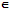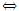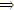# Describe the rings of two sets

Publish On: 2019-03-21

Total Post: 542

# Mjay Jollay

Total Post: 0

## ANS: Describe the rings of two sets

A non-empty set R together with two binary operations ‘*’ and Δ, defined in it, is said to form a ring if the following axioms are satisfied.

(a) Axioms due to the composition “*”:

R1: R is closed w.r.t. “*”, i.e. ∀ a, bRa * bR.

R2: * is associative in R i.e. a * (b * c) = (a * b) * c, ∀ a, b, cR.

R3: Identity element w.r.t. *. There exists eR such that

e * a = a * e = a, for every aR, then

R4: Existence of inverse of every element. For every aR, there exists bR such that a * b = b * a = e, then b is called the inverse of a and vice versa.

R5: * is commutative in R, i.e. a * b = b * a. ∀ a, bR.

(b) Axioms due to both the compositions * a and Δ.

R6: R is closed w.r.t. Δ, i.e. ∀ a, bRa Δ bR.

R7: Δ is associative in R, i.e. a Δ (b Δ c) = (a Δ b) Δ c, ∀ a, b, cR.

R8: Distributive Laws:

Left distributive law: a Δ (b Δ c) = (a Δ b)* (a Δ c), ∀ a, b, cR.

Right distributive law: (b * c) Δ a = (b Δ a)*(c Δ a), ∀ a, b, cR.

In other words, an algebraic structure < R, *, Δ > is said to form an associative ring if

(a) < R, * > is an algebraic group

(b) < R, Δ > is a semi-group

(c) Both right and left distributive laws hold in R.

Some special types of rings

(I) Ring with unity: A ring < R, * Δ > is said to be a ring with unity, if there exists an element gR such that
g Δ a = a = a Δ g                     ∀ aR

then the element g is called the unity of the ring.

(II) Commutative ring: A ring < R, *, Δ > is said to be a commutative ring if Δ is commutative in R
i.e. a Δ b = b Δ a                       ∀ a, bR

(III) Ring without zero divisors: A ring < R, *, Δ > is said to be ring without zero divisors if the product of two non-zero elements or R is zero, i.e. if ∀ a, bR,

a Δ b = 0either a = 0 or b = 0.

(IV) Ring with zero divisors: A ring < R, *, Δ > is said to be ring with zero divisors if for some pair of elements ∀ a, bR, a ≠ 0, b ≠ 0, but, we have

a Δ b = 0.